# PSAT Math : How to find the perimeter of a rectangle

## Example Questions

### Example Question #1 : How To Find The Perimeter Of A Rectangle

A rectangle has a width of 2x. If the length is five more than 150% of the width, what is the perimeter of the rectangle?

6x2 + 5

5x + 5

5x + 10

10(x + 1)

6x2 + 10x

10(x + 1)

Explanation:

Given that w = 2x and l = 1.5w + 5, a substitution will show that l = 1.5(2x) + 5 = 3x + 5.

P = 2w + 2l = 2(2x) + 2(3x + 5) = 4x + 6x + 10 = 10x + 10 = 10(x + 1)

### Example Question #11 : Rectangles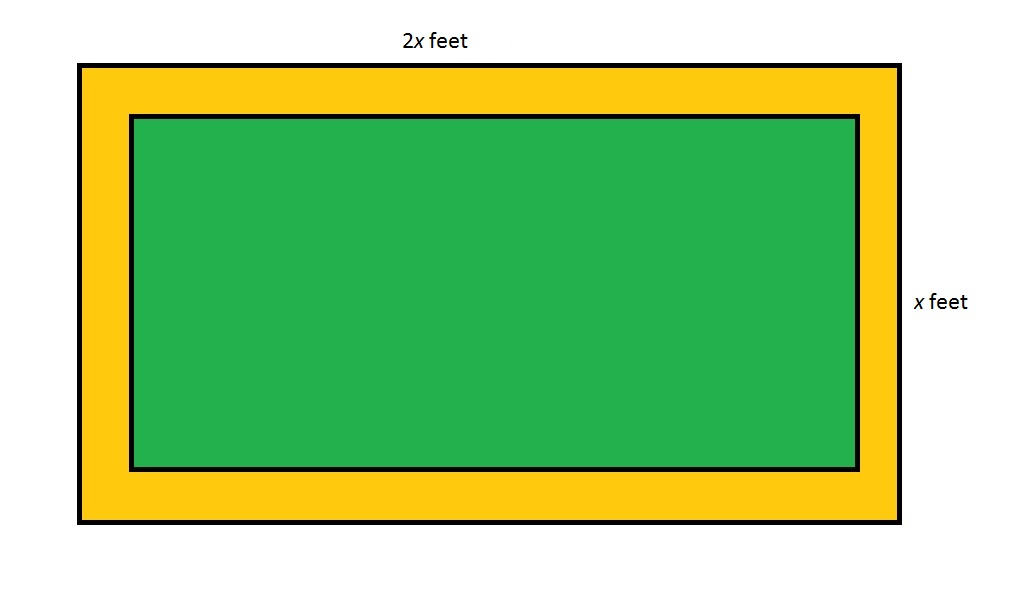Note: Figure NOT drawn to scale

Refer to the above figure, which shows a rectangular garden (in green) surrounded by a dirt path (in orange). The dirt path is seven feet wide throughout. Which of the following polynomials gives the perimeter of the garden in feet?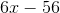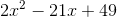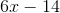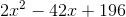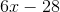Explanation:

The length of the garden, in feet, is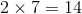feet less than that of the entire lot, or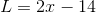;

The width of the garden, in feet, isless than that of the entire lot, or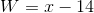.

The perimeter, in feet, is twice the sum of the two: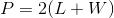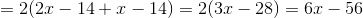### Example Question #12 : Rectangles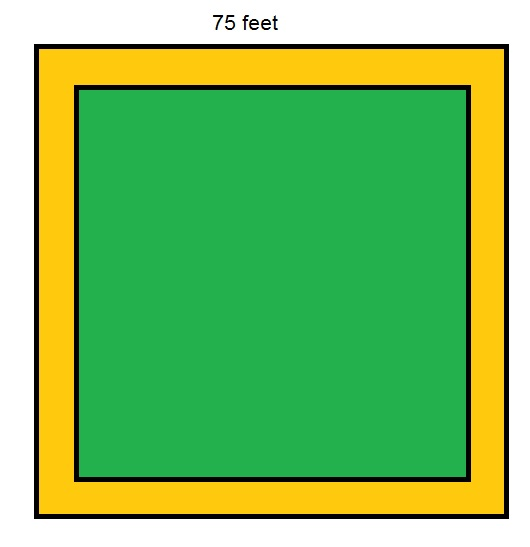Note:  Figure NOT drawn to scale

Refer to the above figure, which shows a square garden (in green) surrounded by a dirt path (in orange) eight feet wide throughout. What is the perimeter of the garden?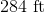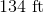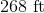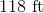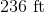Explanation:

The inner square, which represents the garden, has sidelength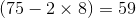feet, so its perimeter is four times this: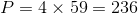feet.

### Example Question #13 : Rectangles

Farmer Dave has a rectangular field that is 50 yards wide and 40 yards long. He wants to enclose the field with a wire fence. How much wire does Farmer Dave need?

160 yards

210 yards

170 yards

200 yards

180 yards

180 yards

Explanation:

To solve this problem, find the perimeter of the rectangle. There are two sides that each measure 50 yards and two sides that each measure 40 yards. Together these four sides measure 180 yards.

### Example Question #6 : How To Find The Perimeter Of A Rectangle

A rectangular garden has an area of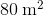. Its length ismeters longer than its width. How much fencing is needed to enclose the garden?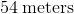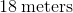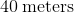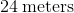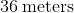Explanation:

We define the variables as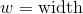and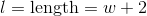.

We substitute these values into the equation for the area of a rectangle and get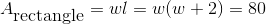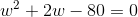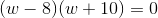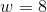or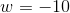Lengths cannot be negative, so the only correct answer is. If, then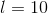Therefore,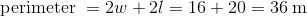.